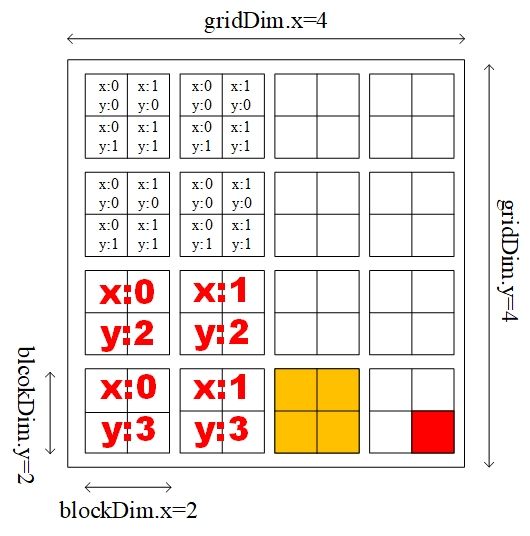### 看图说来12345 const unsigned int idx = threadIdx.x + blockDim.x * blockIdx.x; const unsigned int idy = threadIdx.y + blockDim.y * blockIdx.y; //tid即thread的全局ID const unsigned int tid = idx + blockDim.x * gridDim.x * idy; 
 123456789101112131415 The best way to understand these values is to look at some of the schematics in the Introduction to CUDA Programming document, but I'll an explanation a shot. Basically threadIdx.x and threadIdx.y are the numbers associated with each thread within a block. Let's say you declare your block size to be one dimensional with a size of 8 threads (normally you would want something in multiples of 32 like 192 or 256 depending on your specific code). The variable threadIdx.x would be simultaneously 0,1,2,3,4,5,6 and 7 inside each block. If you declared a two dimensional block size (say (3,3) ) then threadIdx.x would be 0,1,2 and you would now have a threadIdx.y value corresponding to 0,1,2. There are actually nine threads associated with the (3,3) block size. For instance, the thread indices (0,0) (0,1) (1,2) etc refer to independent threads. This convention is very useful for two dimensional applications like working with matrices. Remember, threadIdx.x starts at 0 for each block. Your block can be up to three dimensions which allows for a threadIdx.z index as well. The blockIdx.x and blockIdx.y refers to the label associated with a block in a grid. You are allowed up to a 2-dimensional grid (allowing for blockIdx.x and blockIdx.y). Basically, the blockIdx.x variable is similar to the thread index except it refers to the number associated with the block. Let's say you want 2 blocks in a 1D grid with 5 threads in each block. Your threadIdx.x would be 0, 1,.....,4 for each block and your blockIdx.x would be 0 and 1 depending on the specific block. Now, let's say you want to load an array of 10 values into a kernel using these two blocks of 5 threads. How would you do this since your thread index only goes 0 - 4 for each block? You would use a third parameter given in CUDA -- blockDim.x. This holds the size of the block (in this case blockDim.x = 5). You can refer to the specific element in the array by saying something like... int idx = blockDim.x*blockIdx.x + threadIdx.x This makes idx = 0,1,2,3,4 for the first block because blockIdx.x for the first block is 0. The second block picks up where the first left off because blockIdx.x = 1 and blockDim.x = 5. This makes idx = 5,6,7,8,9 for the second block. Once again, refer to the beginner manual for more on this subject. Hope this helps.